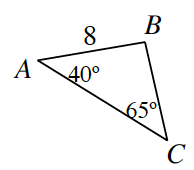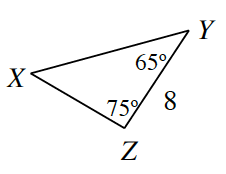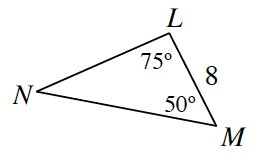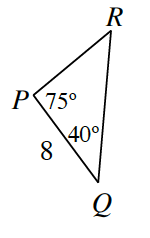### Home > INT2 > Chapter 3 > Lesson 3.1.2 > Problem3-22

3-22.

Refer to $∆ABC$ at right. Determine if each triangle below is congruent to $∆ABC$, similar but not congruent to $∆ABC$, or neither. Justify each answer. If you decide that they are congruent, organize your reasoning into a flowchart.1.Find the missing angles using the Triangle Angle Sum Theorem.

Similar because of $AA\sim$, but not congruent. Why?

1.Not similar and not congruent because there are no corresponding congruent angles between the triangles.

1.